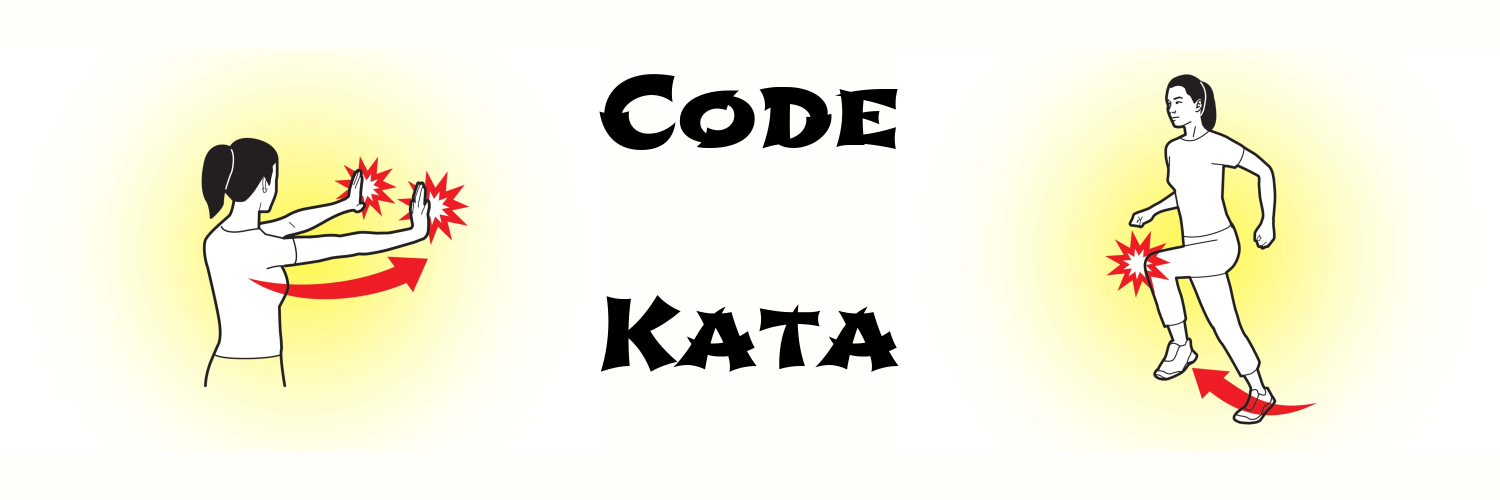programming kata day 10Problem

Here is a list of students’ exam scores. Write a function to sort them based on given criteria. Like Math first, then Physics and then chemistry and then English.

(def exam-scores [{:math 78 :physics 80 :english 97 :chemistry 65}
{:math 78 :physics 80 :english 66 :chemistry 65}
{:math 78 :physics 54 :english 97 :chemistry 65}
{:math 78 :physics 80 :english 97 :chemistry 61}
{:math 100 :physics 89 :english 47 :chemistry 85}
{:math 98 :physics 80 :english 79 :chemistry 65}])

Solution

(defn rank [scores & criteria]
(reverse
(sort-by
(fn [score] (mapv score criteria)) scores)))

Note

The sort-by in Clojure is very powerful, the idea is to reduce each row into one value that we know how to sort, like numbers, strings or lists.